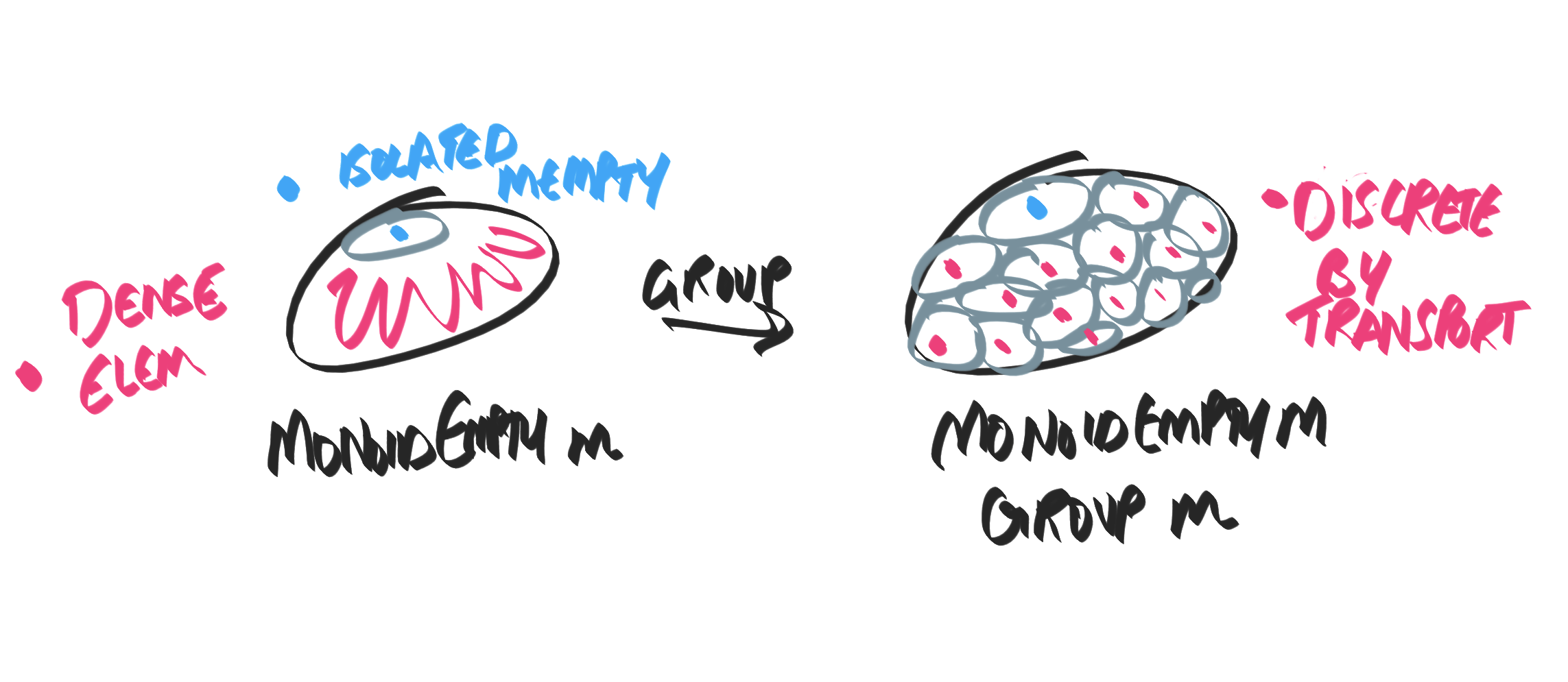## § Topological groups and languages

MonoidNull is a monoid that allow us to test for mempty. So it obeys the law:
class Monoid m => MonoidNull m where
null :: Monoid m => m -> Bool
-- null x == x == mempty

There are MonoidNulls that don't have an Eq instance. For example, consider Maybe (Int -> Int), where the monoid over (Int -> Int) adds them pointwise. Clearly, we can't tell when two functions are equal, so there's no way we can give an Eq instance to Maybe (Int -> Int). But we can definitely tell when it's Nothing! So we have a MonoidNull instance without an Eq instance. Now the interesting thing is that if we have a group that has MonoidNull, then it automatically has Eq! Witness:
instance (Group g, MonoidNull g) => Eq g where
x == y = null (x <> inv y)

See that this is a transport principle: we're able to transport the test of equality at the origin/ mempty provided by null to any point in the group. Olaf Klinke remarked:
A beautiful example of topological groups: Their topology is completely determined by the neighbourhoods of the identity element. If the identity element is isolated, the entire group is discrete.
I found this very interesting, because he's vieweing this from the "topology of computation" lens, where the existence of null means that the identity element is isolated. Now since it is a topological group (group operations are continuous since everything is computable!), the isolatedness of the identity transports to all points, giving us a discrete object where equality is decidable! Below is an illustration of how I imagine the situation.